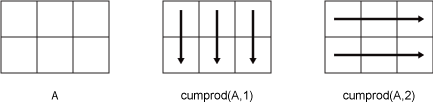# cumprod

Cumulative product

## Syntax

``B = cumprod(A)``
``B = cumprod(A,dim)``
``B = cumprod(___,direction)``
``B = cumprod(___,nanflag)``

## Description

example

````B = cumprod(A)` returns the cumulative product of `A` starting at the beginning of the first array dimension in `A` whose size is greater than 1. If `A` is a vector, then `B` is a vector of the same size containing the cumulative product of `A`.If `A` is a matrix, then `B` is a matrix of the same size containing the cumulative product in each column of `A`.If `A` is a multidimensional array, then `B` is an array of the same size containing the cumulative product along the first array dimension of `A` whose size is greater than 1.If `A` is a table or timetable, then `M` is a table or timetable of the same size containing the cumulative product in each variable of `A`. (since R2023a) The class of `B` is the same as the class of `A` except if `A` is `logical`, in which case `B` is `double`.```

example

````B = cumprod(A,dim)` returns the cumulative product of the elements along dimension `dim`. For example, if `A` is a matrix, then `cumprod(A,2)` returns the cumulative product along the rows of `A`.```

example

````B = cumprod(___,direction)` specifies the direction for any of the previous syntaxes. For example, `cumprod(A,2,"reverse")` returns the cumulative product within the rows of `A` by working from end to beginning of the second dimension.```

example

````B = cumprod(___,nanflag)` specifies whether to include or omit `NaN` values in `A`. For example, `cumprod(A,"omitnan")` ignores `NaN` values when computing each product. By default, `cumprod` includes `NaN` values.```

## Examples

collapse all

Find the cumulative product of the integers from `1` to `5`. The element `B(2)` is the product of `A(1)` and `A(2)`, while `B(5)` is the product of elements `A(1)` through `A(5)`.

```A = 1:5; B = cumprod(A)```
```B = 1×5 1 2 6 24 120 ```

Create a 3-by-3 matrix whose elements correspond to their linear indices.

`A = [1 4 7; 2 5 8; 3 6 9]`
```A = 3×3 1 4 7 2 5 8 3 6 9 ```

Find the cumulative product of the columns of `A`. The element `B(5)` is the product of `A(4)` and `A(5)`, while `B(9)` is the product of `A(7)`, `A(8)`, and `A(9)`.

`B = cumprod(A)`
```B = 3×3 1 4 7 2 20 56 6 120 504 ```

Create a 2-by-3 matrix whose elements correspond to their linear indices.

`A = [1 3 5; 2 4 6]`
```A = 2×3 1 3 5 2 4 6 ```

Find the cumulative product of the rows of `A`. The element `B(3)` is the product of `A(1)` and `A(3)`, while `B(5)` is the product of `A(1)`, `A(3)`, and `A(5)`.

`B = cumprod(A,2)`
```B = 2×3 1 3 15 2 8 48 ```

Create an array of logical values.

`A = [true false true; true true false]`
```A = 2x3 logical array 1 0 1 1 1 0 ```

Find the cumulative product of the rows of `A`.

`B = cumprod(A,2)`
```B = 2×3 1 0 0 1 1 0 ```

The output has type `double`.

`class(B)`
```ans = 'double' ```

Create a 3-by-3 matrix of random integers between 1 and 10.

```rng default; A = randi([1,10],3)```
```A = 3×3 9 10 3 10 7 6 2 1 10 ```

Calculate the cumulative product along the columns. Specify the `"reverse"` option to work from bottom to top in each column. The result is the same size as `A`.

`B = cumprod(A,"reverse")`
```B = 3×3 180 70 180 20 7 60 2 1 10 ```

Create a matrix containing `NaN` values.

`A = [3 5 NaN 4; 2 6 NaN 9; 1 3 0 NaN]`
```A = 3×4 3 5 NaN 4 2 6 NaN 9 1 3 0 NaN ```

Compute the cumulative products of the matrix, excluding `NaN` values. For matrix columns that contain leading `NaN` values, the cumulative product is 1 until a non-`NaN` value is encountered.

`B = cumprod(A,"omitnan")`
```B = 3×4 3 5 1 4 6 30 1 36 6 90 0 36 ```

## Input Arguments

collapse all

Input array, specified as a vector, matrix, multidimensional array, table, or timetable.

Data Types: `double` | `single` | `int8` | `int16` | `int32` | `int64` | `uint8` | `uint16` | `uint32` | `uint64` | `logical` | `table` | `timetable`
Complex Number Support: Yes

Dimension to operate along, specified as a positive integer scalar. If you do not specify the dimension, then the default is the first array dimension of size greater than 1.

Consider a two-dimensional input array, `A`.

• `cumprod(A,1)` works on successive elements in the columns of `A` and returns the cumulative products of each column.

• `cumprod(A,2)` works on successive elements in the rows of `A` and returns the cumulative products of each row.`cumprod` returns `A` if `dim` is greater than `ndims(A)`.

Direction of cumulation, specified as one of these values:

• `"forward"` — Work from `1` to `end` of the operating dimension.

• `"reverse"` — Work from `end` to `1` of the operating dimension.

Missing value condition, specified as one of these values:

• `"includemissing"` or `"includenan"` — Include `NaN` values in `A` when computing the cumulative products. Elements in `B` are `NaN` as soon as the first `NaN` value in `A` is encountered. `"includemissing"` and `"includenan"` have the same behavior.

• `"omitmissing"` or `"omitnan"` — Ignore `NaN` values in `A` when computing the cumulative products. If `A` has consecutive leading `NaN` values, then the corresponding elements in `B` are 1. `"omitmissing"` and `"omitnan"` have the same behavior.

## Tips

• The `"reverse"` option in many cumulative functions allows quick directional calculations without requiring a flip or reflection of the input array.

## Version History

Introduced before R2006a

expand all